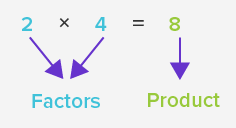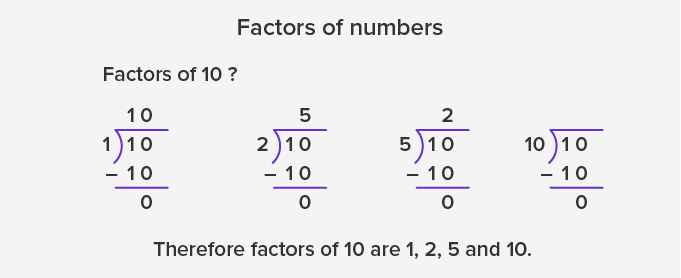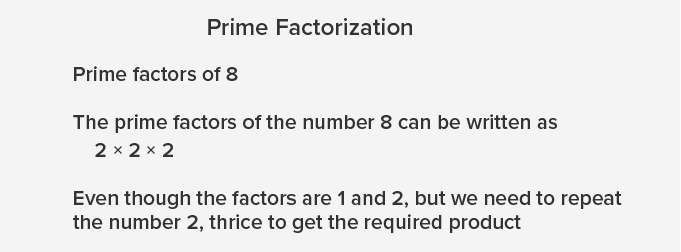Factor - Definition with Examples

The Complete K-5 Math Learning Program Built for Your Child

• 30 Million Kids

Loved by kids and parent worldwide

• 50,000 Schools

Trusted by teachers across schools

• Comprehensive Curriculum

Aligned to Common Core

What is a Factors?

Multiplying two whole numbers gives a product. The numbers that we multiply are the factors of the product.Example:  3 × 5 = 15 therefore, 3 and 5 are the factors of 15.

This also means:

A factor divides a number completely without leaving any remainder.For example:  30 ÷ 6 = 5, and there is no remainder. So we can say that 5 and 6 are the factors of 30.

In the given example, we can further break up or simplify the number 6 into its factors, that is, 2 and 3. In other words, when we multiply 5, 2 and 3, we still get 30. Therefore, the factors of 30 are 5, 2, and 3. Also, 5 × 2 = 10. So, 10 is also a factor of 30. Similarly, 5 × 3 = 15. So, 15 is also a factor of 30. Finally, the factors of 30 are 1, 2, 3, 5, 6, 10, 15 and 30

• The number 1 is the smallest factor of every number.

• Every number will have a minimum of two factors, 1 and the number itself.

• A number that has only two factors, 1 and the number itself, is called a prime number.

Prime factorization

• When we write a number as a product of all its prime factors, it is called prime factorization.

• Every number in prime factorization is a prime number.

• Sometimes to write the prime factors of a number we might have to repeat a number.Fun Facts Factors are always whole numbers or integers and never decimals or fractions. All even numbers will have number 2 as their factor. All numbers that end with 5 will have 5 as their factor. All numbers greater than 0 and ending with a 0 will have 2, 5, and 10 as their factors. Algebraic expressions are often solved or simplified through factoring.

Won Numerous Awards & Honors